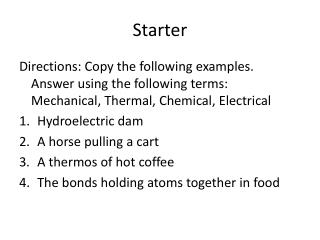DownloadDownload PresentationStarter

# Starter

Download Presentation## Starter

- - - - - - - - - - - - - - - - - - - - - - - - - - - E N D - - - - - - - - - - - - - - - - - - - - - - - - - - -
##### Presentation Transcript

1. Starter Directions: Copy the following examples. Answer using the following terms: Mechanical, Thermal, Chemical, Electrical • Hydroelectric dam • A horse pulling a cart • A thermos of hot coffee • The bonds holding atoms together in food

2. Review • Directions: Complete the statements below. • What is the formula for work? • What is the formula for power? • What is the work if a horse pulls a box 5m with a force of 10 N? • It takes 8 seconds for a pulley system to lift a load with 400 N.m. How much power is required?

3. Review Directions: Draw the following table. Match the example up with the correct simple machine type: Wedge, Lever, Wheel and Axle`, Pulley Example Type • Shovel • Axe • Doorknob • Window blinds

4. What Energy Form? • Directions: Label the picture with the correct energy form. Electrical, nuclear, Thermal, and Mechanical a. b. c. d.

5. Energy Transformation

6. Directions: Each object starts with electrical energy but it converts that energy into another energy. What energy type does it convert into? Thermal, Mechanical, or Electromagnetic

7. Kinetic vs. Potential Kinetic energy Potential Energy Stored (Has the potential to move) The higher the object the greater the potential energy. PE=m(g)(h) PE=Potential m= mass g= gravity h= height Unit=Joule • Motion • The closer the object is to the ground the greater the kinetic energy. • KE=1/2(m)v2 KE= kinetic m= mass v= velocity Unit=Joule

8. The ball starts at rest waiting (PE) to move. Then with a force it moves down the hill (KE).

9. If an object has PE it will have KE. You cannot have one without the other.

10. KE and PE http://www.teachersdomain.org/resource/hew06.sci.phys.maf.rollercoaster/

11. Exit Slip Directions: Copy the examples and label which one is KE or PE. 1. a moving skateboard 2. a dry cell of a battery 3. a rock at the edge of a cliff 4. an acorn hanging from an oak tree 5. a glass of milk 6. a person climbing a ladder 7. Gasoline 8. a piece of celery 9. a basketball passing through a hoop 10. blowing wind